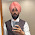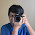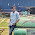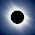# How to Check is a String is Palindrome in Java using Recursion

In this tutorial, you will learn how to check if a string is a palindrome in Java using recursion. A String is nothing but a collection of characters e.g. "Java" and String literals are encoded in double quotes in Java. A String is said to be a palindrome if the reverse of String is equal to itself e.g. "aba" is a palindrome because the reverse of "aba" is also "aba", but "abc" is not a palindrome because the reverse of "abc" is "cba" which is not equal. Recursion means solving a problem by writing a function which calls itself. In order to check if String is a palindrome in Java, we need a function which can reverse the String. Once you have original and reversed String, all you need to do is check if they are equal to each other or not. If they are equal then String is palindrome or not. You can write this reverse() function by using either for loop or by using recursion.

If you remember, I already shared logic of reversing String in my earlier post,  how to reverse String in Java using Iteration and recursion. Here we will use the same logic to check if String is palindrome or not.

By the way, if you are preparing for coding interviews and looking for some coding problem to get hands on practice, I suggest you to take a look at Cracking the Coding Interview: 150 Programming Questions and Solutions. This is a wonderful book, which contains lots of easy and medium difficulty level coding problems, which will not only help you to prepare for interview but also develop your programming logic.

## Java Program to check if String is Palindrome Using Recursion

Here is our Java program, which checks if a given String is palindrome or not. Program is simple and here are steps to find palindrome String :

1) Reverse the given String
2) Check if reverse of String is equal to itself, if yes then given String is palindrome.

In our solution, we have a static method isPalindromeString(String text), which accepts a String. It then call reverse(String text) method to reverse this String. This method uses recursion to reverse String. This function first check if given String is null or empty, if yes then it return the same String because they don't require to be reversed.

After this validation, it extract last character of String and pass rest or String using substring() method to this method itself, hence recursive solution. The validation also servers as base case because after every step, String keeps getting reduced and eventually it will become empty, there your function will stop recursion and will use String concatenation to concatenate all character in reverse order. Finally this method returns the reverse of String.

Once call to reverse() returns back, isPalindromeString(String text) uses equals() method to check if reverse of String is equal to original String or not, if yes then it returns true, which also means String is palindrome.

As I said, if you are looking for more coding based problems you can also always check the Cracking the Coding Interview: 150 Programming Questions and Solutions, one of the great book to build coding sense required to clear programming interviews.

### How to check if String is Palindrome in Java using Recursion

```package test;

/**
* Java program to show you how to check if a String is palindrome or not.
* An String is said to be palindrome if it is equal to itself after reversing.
* In this program, you will learn how to check if a string is a palindrome in java using recursion
* and for loop both.
*
* @author Javin
*/
public class PalindromeTest {

public static void main(String args[]) {
System.out.println("Is aaa palindrom?: " + isPalindromString("aaa"));
System.out.println("Is abc palindrom?: " + isPalindromString("abc"));

System.out.println("Is bbbb palindrom?: " + isPalindromString("bbbb"));
System.out.println("Is defg palindrom?: " + isPalindromString("defg"));

}

/**
* Java method to check if given String is Palindrome
* @param text
* @return true if text is palindrome, otherwise false
*/
public static boolean isPalindromString(String text){
String reverse = reverse(text);
if(text.equals(reverse)){
return true;
}

return false;
}

/**
* Java method to reverse String using recursion
* @param input
* @return reversed String of input
*/
public static String reverse(String input){
if(input == null || input.isEmpty()){
return input;
}

return input.charAt(input.length()- 1) + reverse(input.substring(0, input.length() - 1));
}

}

Output
Is aaa palindrom?: true
Is abc palindrom?: false
Is bbbb palindrom?: true
Is defg palindrom?: false```

You can also solve this problem by retrieving character array from String using toCharArray() and using a for loop and StringBuffer. All you need to do is iterate through character array from end to start i.e. from last index to first index and append those character into StringBuffer object.

Once this is done, just call the toString() method of StringBuffer, its your reversed String. Here is how your code will look like :

How to check if String is Palindrome using StringBuffer and For loop

```import java.util.Scanner;

/**
* How to check if String is palindrome in Java
* using StringBuffer and for loop.
*
* @author java67
*/

public class Palindrome{

public static void main(String args[]) {

Scanner reader = new Scanner(System.in);
System.out.println("Please enter a String");
String input = reader.nextLine();

System.out.printf("Is %s a palindrome? : %b %n", input, isPalindrome(input));

System.out.println("Please enter another String");
input = reader.nextLine();

System.out.printf("Is %s a palindrome? : %b %n", input, isPalindrome(input));

reader.close();

}

public static boolean isPalindrome(String input) {
if (input == null || input.isEmpty()) {
return true;
}

char[] array = input.toCharArray();
StringBuilder sb = new StringBuilder(input.length());
for (int i = input.length() - 1; i >= 0; i--) {
sb.append(array[i]);
}

String reverseOfString = sb.toString();

return input.equals(reverseOfString);
}

}
```

That's all about how to check for palindrome in Java. You have learned how to find if a given String is palindrome using recursion as well by using StringBuffer and for loop. More importantly you have done it by developing your own logic and writing your own code i.e. not taking help from third party library. If you want to do, you can write some unit test for our recursive and iterative palindrome functions and see if it works in all conditions including corner cases.

If you like this coding problem and interested to do more coding exercises, you can also check following beginner level programming exercises. This will help to develop your programming logic and how to use basic tools of a programming language e.g. operators, loop, conditional statements, data structure and core library functions.
• How to reverse words in String in Java? (solution)
• 10 points about array in Java (read here)
• 4 ways to sort array in Java (see here)
• How to print array in Java with examples (read here)
• How to compare two arrays in Java (check here)
• How to declare and initialize multi-dimensional array in Java (see here)
• How to remove element from array without using third party library (check here)
• How to find largest and smallest number in an array in Java (read here)
• Difference between array and ArrayList in Java (see here)
• How to convert Array to String in Java (read here)
• How to find two maximum number on integer array in Java (check here)
• How to loop over array in Java (read here)

Further Learning
The Coding Interview Bootcamp: Algorithms + Data Structures
Data Structures and Algorithms: Deep Dive Using Java
Algorithms and Data Structures - Part 1 and 2

#### 15 comments:

1.public static boolean isPalindromString(String input) {
for (int i = 0; i < input.length() / 2; i++)
if (input.charAt(i) != input.charAt(input.length() - i - 1))
return false;
return true;
}

2.private static void palindrome(String string) {
char[] ch = string.toCharArray();
int count =0;
for(int i=0; i< ch.length/2; i++) {
if(ch[i] == ch[ ch.length-1-i]){

count++;
continue;

}else{
count =0;
System.out.println("not palindrome");
}
}

if(count >0) {
System.out.println(" palindrome");
}

}

3.package String;

import java.util.Scanner;

public class Palindrome {

public static void main(String[] args) {
// TODO Auto-generated method stub
Scanner sc= new Scanner(System.in);
System.out.println("Enter your Palindrome String");

String palindrome =sc.nextLine();
int count=0;
int countlength=palindrome.length()-1;

for(int i=0;i<palindrome.length();i++)
{
if(palindrome.charAt(i)==palindrome.charAt(countlength))
{
count++;
}
countlength--;

}
if(count==palindrome.length())
{
System.out.println(" String is palindrome");
}
else
{
System.out.println(" Strin is not palindrome ");
}

}

}

4.public boolean isPalindrome(String str) {
int len = str.length();
char[] chr = str.toCharArray();
for(int i = 0; i<len/2; i++) {
if(chr[i]!=chr[len-1-i]) {
return false;
}
}
return true;
}

5.isPal(String word) {
boolean isPal = false;
int b_index = 0;
int e_index = word.length()-1;
while(b_index < e_index) {
isPal = word.charAt(b_index) == word.charAt(e_index);
b_index++;
e_index--;
}
return isPal;
}

6.private static Boolean palidromeString(String input, int length){
if(input.length()%2==0){
if(input.length()==0){
return true;
}
if(input.charAt(length-1)==input.charAt(length-length)){
return palidromeString(input.substring(input.length()-(input.length())+1,input.length()-1),
input.substring(input.length()-(input.length())+1,input.length()-1).length());
}
}else {
if(input.length()==1){
return true;
}
return palidromeString(input.substring(input.length()-(input.length())+1,input.length()-1),input.substring(input.length()-(input.length())+1
,input.length()-1).length());
}
return false;
}

7.class Ideone
{
static boolean isPalindrome(String str){
String rev="";
for(int i=str.length();i>=0;i--){
rev+=str.charAt(i);

}
System.out.println(rev);
if(str.equals(rev)){
return true;
}

return false;
}

public static void main(String[] args){
String str="madam";
Ideone ii=new Ideone();
ii.isPalindrome(str);
}

}

Why it is giving the runtime error??

8.private static boolean isPalindrome(String s)
{
return s.equals(new StringBuilder(s).reverse().toString());
}

1.Hello Anonymous, you need to solve this problem with your own logic without using reverse() method from Java framework.

9.public static boolean isPalindrome(String input) {
if(input == null || input.length() < 2) {
return true;
}
for(int i = 0, j = input.length() - 1; i < j; i++, j--) {
if(input.charAt(i) != input.charAt(j)) {
return false;
}
}
return true;
}

1.good solution, keep it up.

10.one liner in python:

def checkPalindrom(input):
return input == input[::-1]

11.one line in python

input == input[::-1]

12.please don't teach anyone to reverse strings using recursion, or worse, check if they are a palindrome by reversing and comparing the string, if an interviewer asks me to do this i will tell him its a bad idea, this is ONLY useful for interviews, a sane programmer would never do this.

1.How would a sane programmer write a function for checking a palindrome?